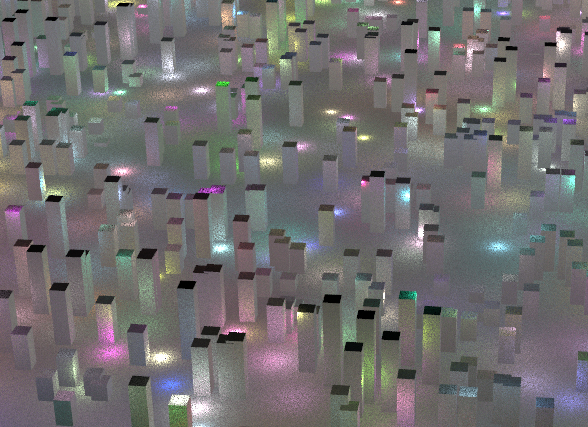## Recommended Posts

Hi there,

I'm trying to instance lights to particles, but I've noticed that object merged lights don't light the scene. Anyone know why that is, or what I'm missing? I've used the instance node, and it works, but I'd really rather use copytopoints if possible.

Thanks,

Matt

##### Share on other sites

@gnarlog ...or...Post that scene Please. I have this code Old, just use on that saved points on Mantra In-line Code .

```\$out = 0;//npoints(\$input);
int size = npoints(\$input);
int samples = 800;
vector pos[];
resize(pos, size);

float cdf[];

for (int i=0; i<npoints(\$input); i++)
{
vector Lpos = ptransform("space:world", "space:camera", point(\$input,"P",i));
pos[i] = Lpos;
cdf[i] = 1/distance2(P,Lpos) * max(0 ,dot(normalize(N), normalize(Lpos-P))) + cdf[i-1];
}

int getI(float cdf[]; float r)
{
int i = 0;
r*=cdf[-1];
while(r<cdf[i]) i++;
return i;
}

for (int i=0;i<samples;i++){

int id = int(nrandom("qstrat")*size);
vector Lpos = pos[id];

\$out += vector(point(\$input,"Cd",id))/distance2(P,Lpos) * max(0, dot(normalize(N), normalize(Lpos-P)));
}

\$out /= samples;```##### Share on other sites
1 hour ago, Librarian said:

@gnarlog ...or...Post that scene Please. I have this code Old, just use on that saved points on Mantra In-line Code .

```
\$out = 0;//npoints(\$input);
int size = npoints(\$input);
int samples = 800;
vector pos[];
resize(pos, size);

float cdf[];

for (int i=0; i<npoints(\$input); i++)
{
vector Lpos = ptransform("space:world", "space:camera", point(\$input,"P",i));
pos[i] = Lpos;
cdf[i] = 1/distance2(P,Lpos) * max(0 ,dot(normalize(N), normalize(Lpos-P))) + cdf[i-1];
}

int getI(float cdf[]; float r)
{
int i = 0;
r*=cdf[-1];
while(r<cdf[i]) i++;
return i;
}

for (int i=0;i<samples;i++){

int id = int(nrandom("qstrat")*size);
vector Lpos = pos[id];

\$out += vector(point(\$input,"Cd",id))/distance2(P,Lpos) * max(0, dot(normalize(N), normalize(Lpos-P)));
}

\$out /= samples;```

Oh, wow, cool! Thank you! So I take it lights have to be manipulated at the object level? I'll post a scene here for you to look at...

Thanks a lot for taking the time to do this!

light_instance_test_v001.hip

##### Share on other sites

Just got a chance to take a look at this.

Wow, I wasn't even aware of this method, but it's really neat! I'm not  a coder, so pardon me if this is a dumb questions but:

How is it that \$out is initialized to 0, but later in a for loop is having a vector added to it?

Might you have an example hip file?

Thank you again for sharing this.

## Create an account

Register a new account